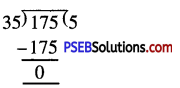# PSEB 4th Class Maths Solutions Chapter 2 Fundamental Operations on Numbers Ex 2.9

Punjab State Board PSEB 4th Class Maths Book Solutions Chapter 2 Fundamental Operations on Numbers Ex 2.9 Textbook Exercise Questions and Answers.

## PSEB Solutions for Class 4 Maths Chapter 2 Fundamental Operations on Numbers Ex 2.9

Question 1.
In morning assembly 161 students stand in 7 rows equally. How many students stand in each row ?
Solution:
Total number of student = 161
Number of rows = 7
Number of students in each row = 161 ÷ 7 = 23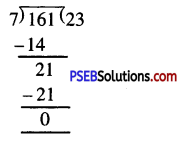Question 2.
I have 72 apples I have to put these in 3 buckets equally. How many apples each basket will contain ?
Solution:
The total number of apples = 72
Number of apples in each basket = 72 ÷ 3 = 24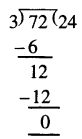Question 3.
A farmer has produced 4250 kg wheat in his Held. One bag is required to fill for 50 kg of wheat. How many such bags are required to fill 4250 kg wheat ?
Solution:
Total quantity of wheat produced = 4250 kg
Quantity of wheat in one bag = 50 kg
Number of bags required = 4250 ÷ 50 = 85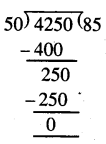Question 4.
By which number to multiply 25 so that product becomes 625 ?
Solution:
Product of the two numbers = 625
One of the number = 25 Second number = 625 ÷ 25 = 25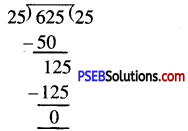Question 5.
A gardener has 120 flowers. He has to make garland of 24 flowers. How many such garlands are made from 120 flowers ?
Solution:
The total number of flowers = 120
Number of flowers in 1 garland = 24
Number of garlands that can be made = 120 ÷ 24 = 5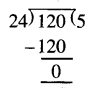Question 6.
How many ₹ 50 notes are required to make ₹ 2000 ?
Solution:
Total amount = ₹ 2000
Value of 1 note = ₹ 50
Number of notes required = ₹ 2000 ÷ ₹ 50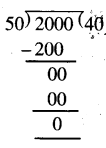Question 7.
I want to exchange my ₹ 500 note. How many notes of following denomination will I get ?
(a) If all are ₹ 100 notes …………………….
(b) If all are ₹ 50 notes ……………………
(c) If all are ₹ 10 notes ……………………..
Solution:
(a) If all are ₹ 100 notes then ₹ 500 ÷ ₹ 100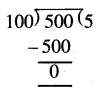= 5 notes are required to exchange ₹ 500 note.

(b) If all are ₹ 50 notes then ₹ 500 ÷ ₹ 50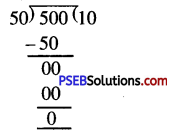= 10 notes are required to exchange
= ₹ 500 note

(c) If all are ₹ 10 notes then ₹ 500 ÷ ₹ 10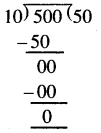= 50 notes are required to exchange a ₹ 500

Question 8.
A labourer picks up 20 bricks in 1 round. How many number of rounds are required to pick up 1000 bricks ?
Solution:
Total number of bricks = 1000
Number of bricks picked in 1 round = 20
Number of rounds = 1000 ÷ 20 = 50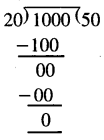Question 9.
A railway ticket costs ₹ 24. palak gave ₹ 576 to the Station Master. How many mumber of tickets did she get ?
Solution:
The cost of 1 ticket = ₹ 24
Palak gave the money = ₹ 576
No of tickets = ₹ 576 ÷ 24 = 24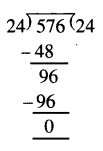Question 10.
Kashvi brought a toffees packet on her birthday. There are 175 toffees in this packet and there are 35 students in her class. How many toffees did each of them get.
Solution:
Total ho. of toffees = 175
No. of children = 35
No. of toffees that each child will get = 175 ÷ 35 = 5